##### Basic Math & Pre-Algebra All-in-One For Dummies (+ Chapter Quizzes Online)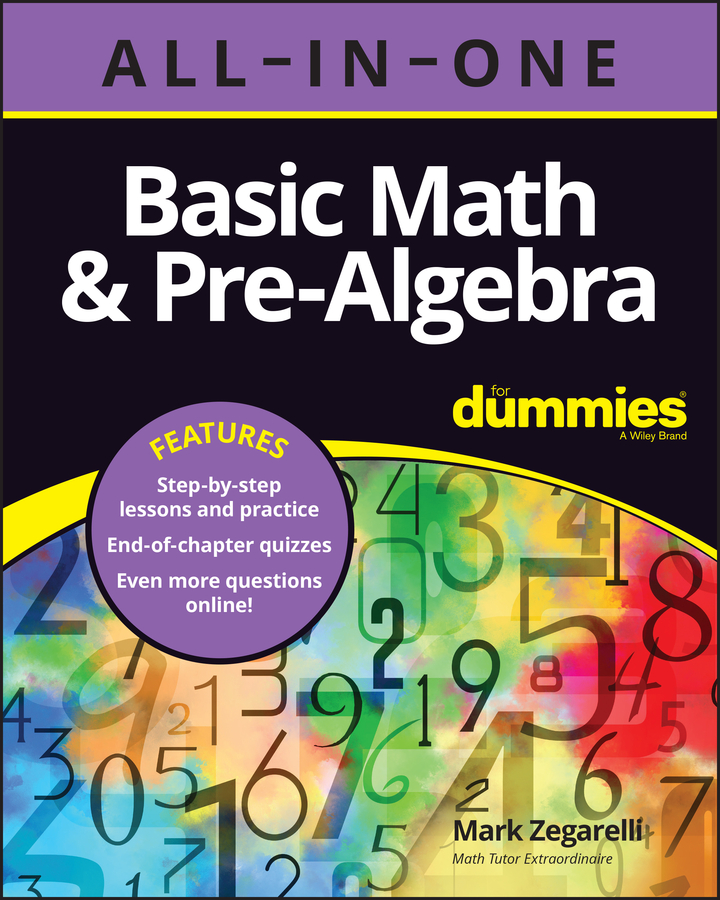At times, monomials can have coefficients and/or be raised to a power before you begin the binomial expansion. In this case, you have to raise the entire monomial to the appropriate power in each step. For example, here's how you expand the expression (3x2 – 2y)7:

1. Write out the binomial expansion by using the binomial theorem, substituting in for the variables where necessary.

In case you forgot, here is the binomial theorem: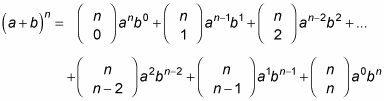Replace the letter a in the theorem with the quantity (3x2) and the letter b with (–2y). Don't let those coefficients or exponents scare you — you're still substituting them into the binomial theorem. Replace n with 7. You end up with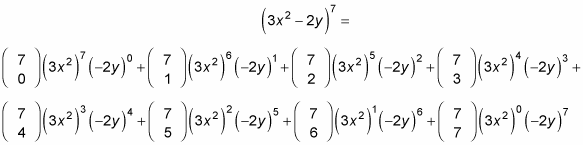2. Find the binomial coefficients.

The formula for binomial expansion is written in the following form: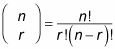You may recall the term factorial from your earlier math classes. If not, here is a reminder: n!, which reads as "n factorial," is defined as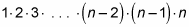Now, back to the problem. Using the combination formula gives you the following: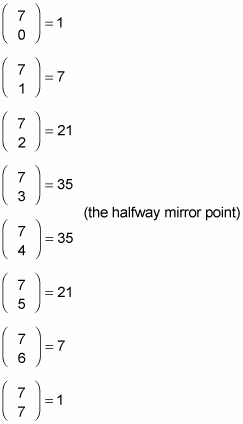3. Replace all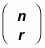4. with the coefficients from Step 2.

1(3x2)7(–2y)0 + 7(3x2)6(–2y)1 + 21(3x2)5(–2y)2 + 35(3x2)4(–2y)3 + 35(3x2)3(–2y)4 + 21(3x2)2(–2y)5 + 7(3x2)1(–2y)6 + 1(3x2)0(–2y)7

5. Raise the monomials to the powers specified for each term.

1(2,187x14)(1) + 7(729x12)(–2y) + 21(243x10)(4y2) + 35(81x8)(–8y3) + 35(27x6)(16y4) + 21(9x4)(–32y5) + 7(3x2)(64y6) + 1(1)(–128y7)

6. Simplify.

2,187x14 – 10,206x12y + 20,412x10y2 – 22,680x8y3 + 15,120x6y4 – 6,048x4y5 + 1,344x2y6 – 128y7